## Trigonometry Values Pi/17

Rather surprisingly, trigonometric functions offoran integer can be expressed in terms of sums, products, and finite root extractions because 17 is a Fermat Prime. This makes the Heptadecagon a Constructible, as first proved by Gauß.Although Gauss did not actually explicitly provide a construction, he did derive the trigonometric formulas below using a series of intermediate variables from which the final expressions were then built up.

Let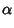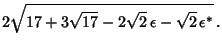Then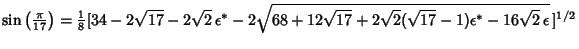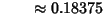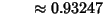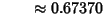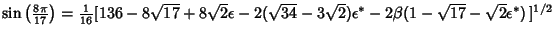There are some interesting analytic formulas involving the trigonometric functions of. Define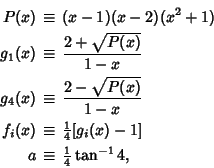whereor 4. Then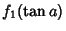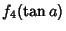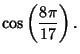References

Casey, J. Plane Trigonometry. Dublin: Hodges, Figgis, & Co., p. 220, 1888.

Conway, J. H. and Guy, R. K. The Book of Numbers. New York: Springer-Verlag, pp. 192-194 and 229-230, 1996.

Dörrie, H. The Regular Heptadecagon.'' §37 in 100 Great Problems of Elementary Mathematics: Their History and Solutions. New York: Dover, pp. 177-184, 1965.

Ore, Ø. Number Theory and Its History. New York: Dover, 1988.

Smith, D. E. A Source Book in Mathematics. New York: Dover, p. 348, 1994.